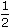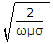# Electronics and Communication Engineering - Electronic Devices and Circuits

1.
Which of these has 3 layers?
PIN diode
Zener diode
Schottky diode
Photo diode
Explanation:

p layer, i layer and n layer.

2.
As per Einstein's equation, the velocity of emitted electron in photoelectric emission is given by the equationmv2 = hf - Uwmv2hf - Uwmv2 = hf + Uwmv2hf + Uw
Explanation:

Some electrons have less energy than Fermi level E and, therefore, more energy than Uw to escape.

Therefore, this equation has inequality sign.

3.
For an P-channel enhancement type MOSFET determine the drain current if K = 0.278 x 10-3A/V2, VGS = -4V, VT = -2V, Voltage equivalent at 27°C = 26 mV.
10 mA
1.11 mA
0.751 mA
46.98 mA
Explanation:

Drain current Id  = k (|Vgs| - |VT|)2

= 0.278 x 10-3(4 - 2)2

= 1.11 mA.

4.
The skin depth of copper is found to be 66 mm at 1 MHz at a certain temperature. At the same temperature and at 2 MHz, the skin depth would be approximately
47 μm
33 μm
92 μm
1.22 μm
Skin depth =